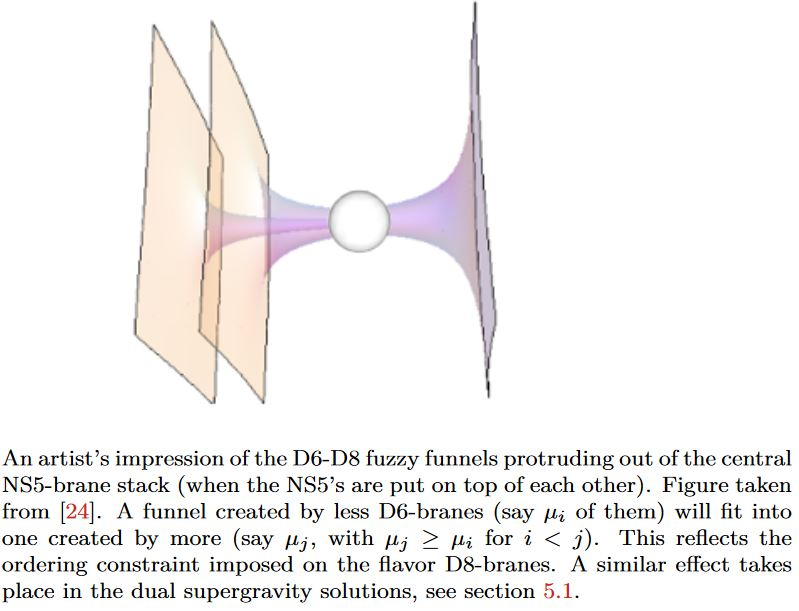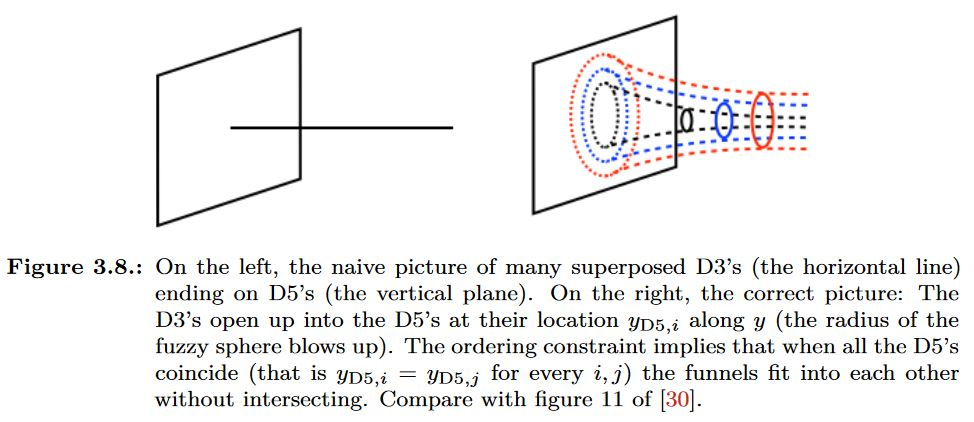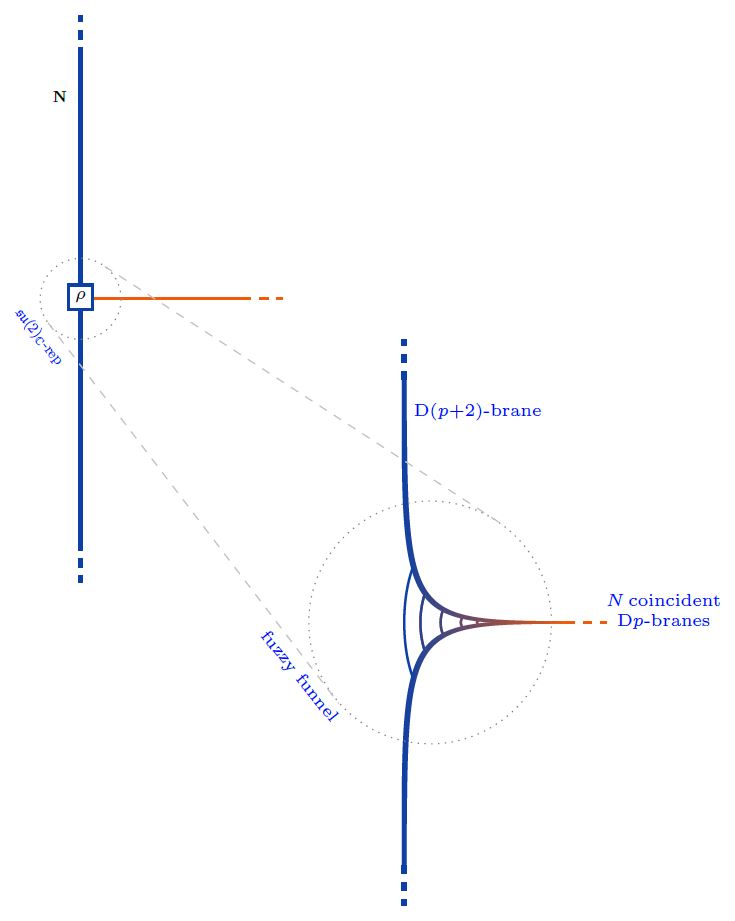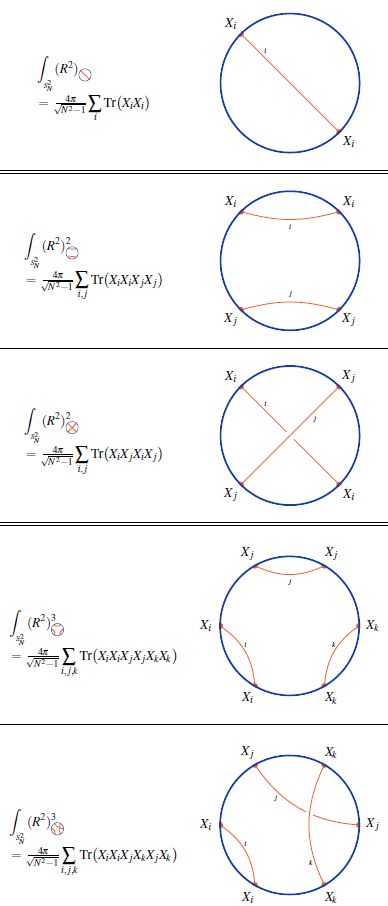Contents

# Contents

## Idea

The microscopic geometry of transversal Dp-D(p+2)-brane intersections and Dp-D(p+4)-brane intersections look like warped non-commutative metric cones on fuzzy spheres (namely on the spheres around the lower dimensional D-branes inside the higher dimensional D-branes). These have hence been called fuzzy funnels.graphics grabbed from Fazzi 17, Fig. 3.14, taken in turn from Gaiotto-Tomassiello 14, Figure 5graphics grabbed from Fazzi 17

### Transveral Dp-D(p+2)-brane intersections in fuzzy funnels

The boundary condition in the nonabelian DBI model of coincident Dp-branes describing their transversal intersection/ending with/on D(p+2)-branes is controled by Nahm's equation and thus exhibits the brane intersection-locus equivalently as:

1. a fuzzy funnel noncommutative geometry interpolating between the $\mathrm{D}p$- and the $\mathrm{D}(p+2)$-brane worldvolumes;

2. geometric engineering of Yang-Mills monopoles in the worldvolume-theory of the ambient $D(p+2)$-branes.

More explicitly, for $y \in (0,\infty ]$ the transversal distance along the stack of $N$ $\mathrm{D}p$-branes away from the $\mathrm{D}(p+2)$-brane, and for

$X^i \in C^\infty\big( (0,\infty], \mathfrak{u}(N) \big) \phantom{AAA} i \in \{1,2,3\}$

the three scalar fields on the worldvolume, the boundary condition is:

$\frac{d}{d y} X^3 + [X^1, X^2] \;=\; 0 \,, \;\;\; \frac{d}{d y} X^1 + [X^2, X^3] \;=\; 0 \,, \;\;\; \frac{d}{d y} X^2 + [X^3, X^1] \;=\; 0$

as $y \to 0$. These are Nahm's equations, solved by

$X^i(y) = \frac{1}{y} \rho^i + \text{non-singular}$

where

$\rho \;\colon\; \mathfrak{su}(2) \longrightarrow \mathfrak{u}(N)$

is a Lie algebra homomorphism from su(2) to the unitary Lie algebra, and

$\rho^i \coloneqq \rho(\sigma^i)$

is its complex-linear combination of values on the canonical Pauli matrix basis.Equivalently. $\rho$ is an $N$-dimensional complex Lie algebra representation of su(2). Any such is reducible as a direct sum of irreducible representations $\mathbf{N}^{(M5)}$, for which there is exactly one, up to isomorphism, in each dimension $N^{(M5)} \in \mathbb{N}$:

(1)$\rho \;\simeq\; \underset{ i }{\bigoplus} \big( N_i^{(M2)} \cdot \mathbf{N}_i^{(M5)} \big) \,.$

(Here the notation follows the discussion at M2/M5-brane bound states in the BMN model, which is the M-theory lift of the present situation).

Now each irrep $\mathbf{N}_i^{(M5)}$ may be interpreted as a fuzzy 2-sphere of radius $\propto \sqrt{ \left( N_i^{(M5)}\right)^2 - 1 }$, hence as the section of a fuzzy funnel at given $y = \epsilon$, whence the totality of (1) represents a system of concentric fuzzy 2-spheres/fuzzy funnels.

graphics from Sati-Schreiber 19c

Moreover, since the complexification of su(2) is the complex special linear Lie algebra $\mathfrak{sl}(2,\mathbb{C})$ (here) the solutions to the boundary conditions are also identified with finite-dimensional $\mathfrak{sl}(2,\mathbb{C})$ Lie algebra representations:

(2)$\rho \;\in\; \mathfrak{sl}(2,\mathbb{C}) Rep \,.$

This is what many authors state, but it is not yet the full picture:

Also the worldvolume Chan-Paton gauge field component $A$ along $y$ participates in the brane intersection

$A \in C^\infty\big( (0,\infty], \mathfrak{u}(N) \big)$

its boundary condition being that

$[A, X^i] \;=\; 0 \phantom{AAAA} \text{for all}\; i \in \{1,2,3\}$

as $y \to 0$ (Constable-Myers 99, Section 3.3, Thomas-Ward 06, p. 16, Gaiotto-Witten 08, Section 3.1.1)

Together with (2) this means that the quadruple of fields $(X^1,X^2,X^3,A)$ constitutes a Lie algebra representation of the general linear Lie algebra

$\mathfrak{gl}(2,\mathbb{C}) \;\simeq\; \underset{ \langle X^1, X^2, X^3 \rangle }{ \underbrace{ \mathfrak{sl}(2,\mathbb{C}) } } \oplus \underset{ \langle A \rangle }{ \underbrace{ \mathbb{C} } }$

This makes little difference as far as bare Lie algebra representations are concerned, but it does make a crucial difference when these are regarded as metric Lie representations of metric Lie algebras, since $\mathfrak{gl}(2,\mathbb{C})$ admits further invariant metrics…

## Properties

### Single trace observables as $\mathfrak{su}(2)$-weight systems on chord diagrams

We discuss how the single trace observables on the fuzzy 2-sphere-sections of Dp-D(p+2) brane intersection fuzzy funnels are given by su(2)-Lie algebra weight systems on chord diagrams (following Ramgoolam-Spence-Thomas 04, McNamara-Papageorgakis 05, see McNamara 06, Section 4 for review).

For more see at weight systems on chord diagrams in physics.While in the commutative large N limit, all powers of the radius function on the fuzzy 2-sphere are equal

$\underset{N\to \infty}{\lim} \int_{S^2_N} R^{2 k} \;=\; 4 \pi \,;$

for finite $N$ there is an ordering ambiguity: In fact, the number of functions on the fuzzy 2-sphere at finite $N$ that all go to the same function $R^{2k}$ in the large N limit grows rapidly with $k$.

At $k = 1$ there is the single radius observable (?)

$\int_{S^2_N} R^2 \;=\; \int_{S^2_N} \underset{i}{\sum} X_i \cdot X_i \;=\; 4 \pi \tfrac{ N }{ \sqrt{N^2 -1} }$

At $k = 2$ there are, under the integral (?), two radius observables:

1. $\int_{S^2_N} \underset{i,j}{\sum} X_i X_i X_j X_j$

2. $\int_{S^2_N} \underset{i,j}{\sum} X_i X_j X_j X_i$

(Here we are using that under the integral/trace, a cyclic permutation of the factors in the integrand does not change the result).

Similarly for higher $k$, where the number of possible orderings increases rapidly. The combinatorics that appears here is familiar in knot theory:

Every ordering of operators, up to cyclic permutation, in the single trace observable $Tr(R^2)^n$ is encoded in a chord diagram and the value of the corresponding single trace observable is the value of the su(2)-Lie algebra weight system on this chord diagram.

brane intersections/bound states/wrapped branes/polarized branes

S-duality$\,$bound states:

intersecting$\,$M-branes:

### General

• Rajsekhar Bhattacharyya, Robert de Mello Koch, Fluctuating Fuzzy Funnels, JHEP 0510 (2005) 036 (arXiv:hep-th/0508131)

### For D1-D3-brane intersections

On D1-D3 brane intersections as fuzzy funnels on fuzzy 2-spheres:

### For D3-D5 brane intersections

On D3-D5 brane intersections as fuzzy funnels on fuzzy 2-spheres:

### For D6-D8 brane intersections

On D6-D8 brane intersections as fuzzy funnels on fuzzy 2-spheres:

### For D1-D5-brane intersections

On D1-D5 brane intersections as fuzzy funnels on fuzzy 4-spheres:

### For D1-D7-brane intersections

On D1-D7 brane intersections as fuzzy funnels on fuzzy 6-spheres:

### Single trace observables as weight systems on chord duagrams

Relation of single trace observables on Dp-D(p+2)-brane bound states (hence Yang-Mills monopoles) to su(2)-Lie algebra weight systems on chord diagrams computing radii averages of fuzzy spheres: